```Calculating Temperatures Under Hood of a Prebake Anode Cell
```

### Calculation of heat contents of gases

The moisture content of the air drawn in has a significant effect on the heat capacity of the air. To get the percent moisture in the ambient air first we calculate the mass % water in water saturated air2 based on the temperature of the air in the potroom.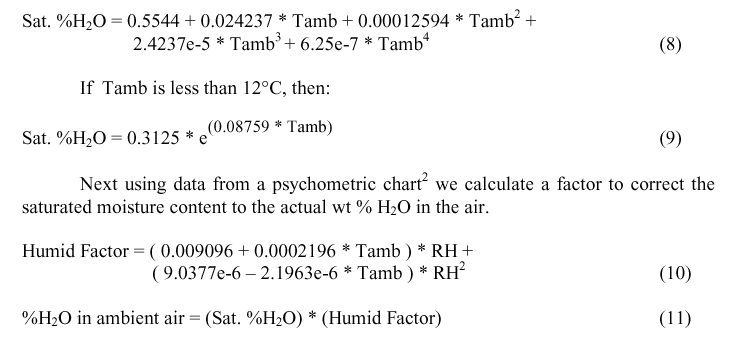### Cp(blend) used to calculate the temperature rise of Qtop, Qcombust and Qairburn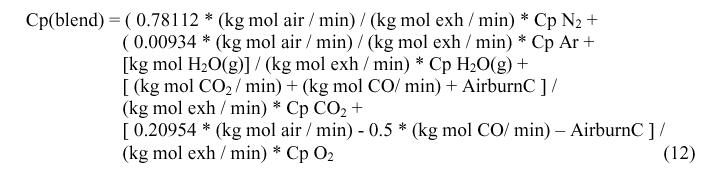### Cp(mix) used to calculate DT rise caused by removing heat from the anode rods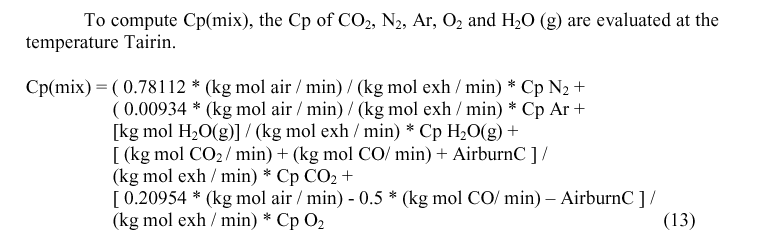### Calculating the cell's evolution of CO and CO2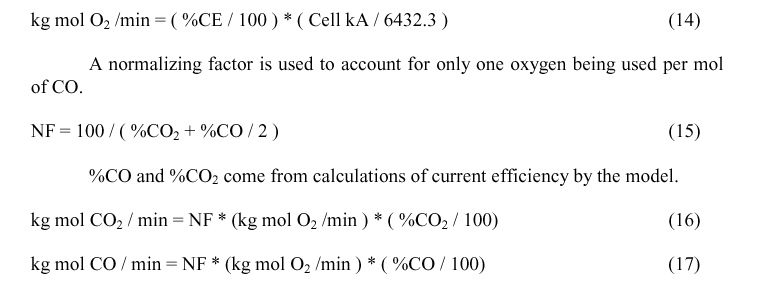### Calculating air drawn into cell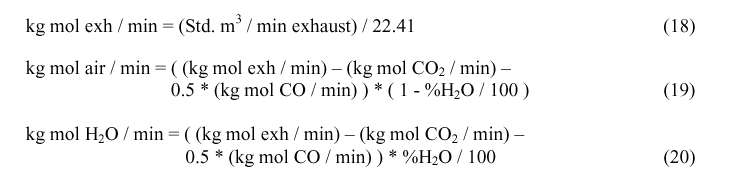### Calculating Tblend### Calculating Tmix

Calculating Qcombust

Qcombust, the quantity of heat generated by the combustion of CO as it exits the electrolyte is needed to calculate Tmix. Qcombust is obtained by calculating the enthalpy of the reaction: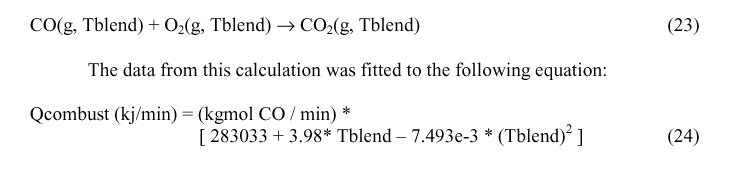Calculating Qairburn

We take the total carbon consumption by electrolysis and multiply it by a fraction representing the amount that air burns to get the airburn carbon. Net carbon consumption will be the sum of electrolytically consumed Carbon plus AirburnC plus Dusting.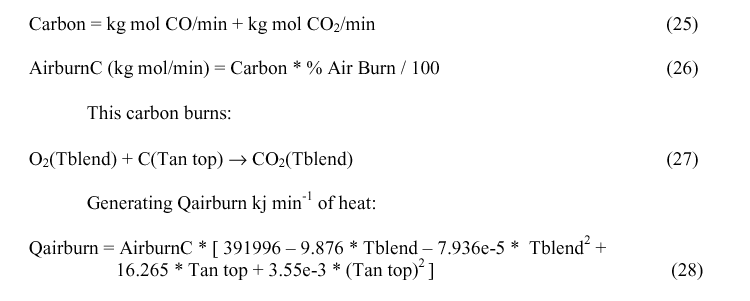Calculating Tmix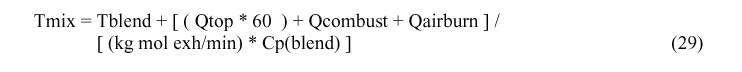### Calculating Texh and Tairin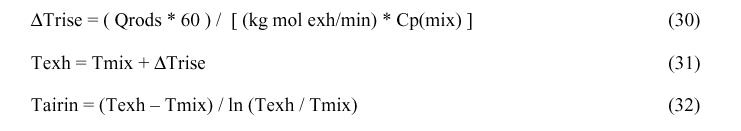Because the calculation of many of the values depend upon Tblend, Tmix and Tairin, not yet calculated, the solution has to be an iterative process. The suggested value for first iteration is Tblend = 50° C, Tmix = 160° C and Tairin = 170° C.

ANALYSIS OF RESULTS

Figure 1 shows, as expected that the temperature under the hood will drop as more air is drawn in.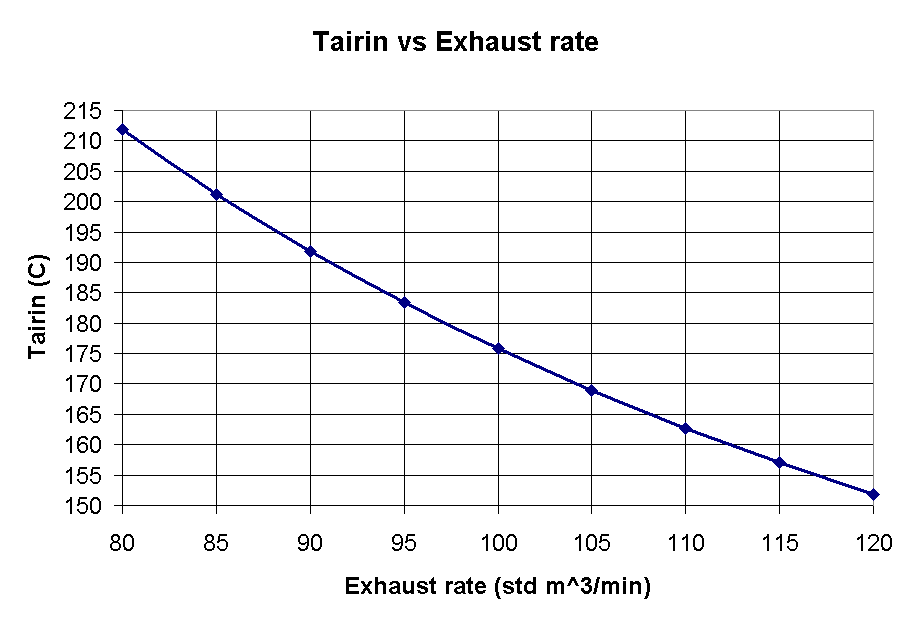Figure 1 - Tairin vs Exhaust rate

Figure 2 indicates that as current efficiency increases, the temperature under the hood should drop. This is an idealized curve and may not be seen in practice. It is true that more energy goes into making aluminum and less into heat at higher current efficiencies. Changes in crust cover and anode changing will often obscure the effect of current efficiency.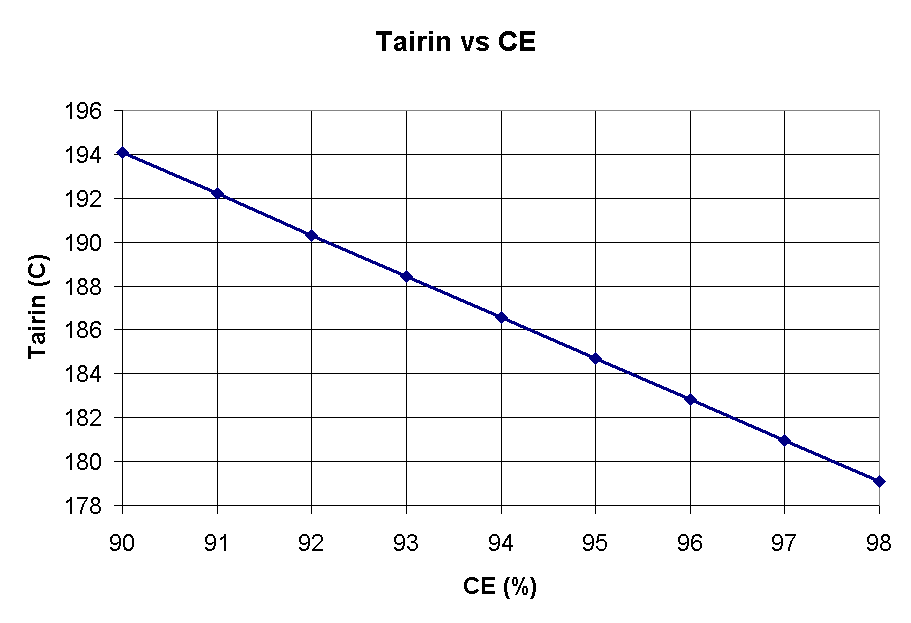Figure 2 - Tairin vs Current efficiency

Figure 3 shows, as expected that the temperature under the hood will increase with increasing heat off the top of the cell.Figure 3 - Tairin vs Sum of heat loss from ore covered and anode stubs

Figure 4 shows that similarly to the current efficiency, the amount of anode carbon that air burns has a non-negligible impact on the temperature under the hood.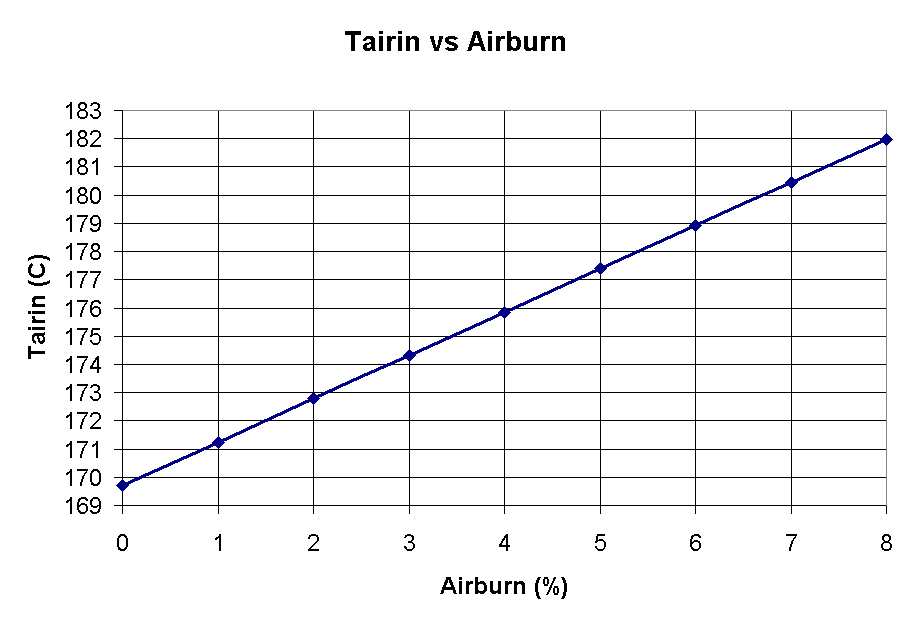Figure 4 - Tairin vs Anode carbon air burn

CONCLUSIONS

A method has been presented to estimate the temperatures found under the hood of a prebaked anode cell. The equations presented in this paper have been programmed into a computer program that will let you estimate the gas temperature distribution under the hood. You can download a free copy of this program from the GeniSim Web site at www.genisim.com/download/tairin.exe.

REFERENCES

1. W.N. Chase Jr, C.A. Davies, J.R. Downey, Jr, D.F. Frurip, R.A. McDonald, and A.N. Syverud, 1985.
JANAF Thermochemical Tables, 3rd Edition, Publised by thre American Chemical Society and the American Institute of Physics for the National Bureau of Standards

2. Perry and Chilton, 1973.
Chemical Engineers' Handbook, Fifth Edition, McGraw-Hill, New York, Pages 12-5, 12-7 and 12-8.